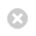Close

UCL Module Catalogue

Home# Numerical Methods for Earth Sciences (GEOL0018)

## Key information

Faculty
Faculty of Mathematical and Physical Sciences
Teaching department
Earth Sciences
Credit value
15
Restrictions
Prerequisites for this module are PHAS0002 Maths Methods I, PHAS0009 Maths Methods II or equivalent Maths modules with permission from the module organiser.

## Alternative credit options

There are no alternative credit options available for this module.

## Description

This module covers the basic principles of numerical and computational methods to solve geophysical problems. It is focused mostly around the use of finite differences to solve differential equations. After an introduction to the concept of numerical solutions to mathematical equations, the course will detail the concept of finite differences (and variations around them) to solve initial value problems and boundary value problems for Ordinary Differential Equations (ODE), and Partial Differential Equations (PDE). After the basics are covered, a series of applications will be presented, covering a range of applied geophysical problems.

By the end of the module students should:

• Understand how to discretsize and compute solutions to simplified differential equations;
• Understand the meaing and accuracy of the numerical solution and benchmarking techniques;
• Have a solid base of computational skills and be able to write their own codes and present their results from scratch using Matlab.

Prerequisites for this module are PHAS0002 Maths Methods I, PHAS0009 Maths Methods II or equivalent Maths modules with permission from the module organiser.

## Intended teaching term: Term 2     Undergraduate (FHEQ Level 5)

### Teaching and assessment

Mode of study
Face-to-face
Methods of assessment
40% Coursework
60% In-class Exam (2 hours)
Mark scheme
Numeric Marks

### Other information

Number of students on module in previous year
10